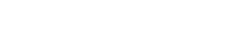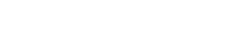# Unit Cost Method of Estimation in Construction ProjectsReading time: 1 minute

Unit cost method of estimation in construction projects starts with dividing a project into various components or elements for the purpose of cost estimation. The cost of each of the project's components or elements are assessed and their cost estimation is calculated. Sum of costs of each project elements gives the total construction cost of the project. The unit cost method of estimation can be used for project design estimates as well as for bid estimates. Construction cost estimates are of three types mentioned below:
1. Preliminary Cost Estimates
2. Detailed Cost Estimates
3. Engineer's Cost Estimates
During the preliminery cost estimate, as cost details of every minute elements are not of much importance, the project is divided into major components and cost estimate of these major units are calcualted based on the past project experiences. The cost of euipments if any is also included. For example, estimating the cost of entire floor based on its area. During detailed Cost estimates, the project is divided into components of various major systams and cost of each components is estimated to calculate project cost. For example, cost of installation of any equipment, cost of beams, columns or walls etc. During Engineer's cost estimates, each of the components of the major systems of the project is divided into various components that contributes to the cost of the major systems. For example, for cost estimation of an RCC column, the components are concrete, which is also divided into cement, sand and aggregates, reinforcement steel, formworks etc. The unit cost of each of these materials are considered by calculating the cost of an RCC column.For bid estimates, the unit cost method of estimation can also be used even though the contractor divides the project into different levels in a hierarchy as follows:
1. Subcontractor Quotations: The general contractor of the construction project may get quotations for various items from the subcontractors. The rates prescribed by the subcontractors for various items of the projects can be used for the total construction cost etimate for the project. However, the reliability of accuracy of the cost depends on the method of cost estimates selected by the subcontractor.
2. Quantity Takeoffs: The division of a construction project into various items of quantities that are measured from the engineer's plan will result in a procedure similar to that adopted for a detailed estimate or an engineer's estimate by the design professional. The levels of detail may vary according to the desire of the general contractor and the availability of cost data.
3. Construction Procedures: If the construction procedure of a proposed project is used as the basis of a cost estimate, the project may be decomposed into items such as labor, material and equipment needed to perform various tasks in the projects.

## Formula for Unit Cost Method

Suppose that a construction project is divided into "n" elements for cost estimation. Let "Qi" be the quantity of the ith element and ui be the corresponding unit cost. Then, the total cost of the project is given by:where n is the number of units. The unit cost of element ui is estimated based on the method of construction, technology used, type of materials used etc.

### Factored Unit Cost Estimate Formula

Sometimes during the cost estimate, there can be some of the minute components which may not have been considered or there can be some variations w.r.t time. In such cases, the cost of units are factored as an allowance for variations or the elements not considered in the estimates. The formula for factored unit cost estimation is as follows:where Ci is the purchase cost of a major component i and fi be a factor accounting for the cost variation of the item, n is the number of components included in the construction project.

### Formula Based on Labor, Material and Equipment

Suppose that a construction project is decomposed into n tasks. Let Qi be the quantity of work for task i, Mi be the unit material cost of task i, Ei be the unit equipment rate for task i, Li be the units of labor required per unit of Qi, and Wi be the wage rate associated with Li. In this case, the total cost y is:Note that WiLi yields the labor cost per unit of Qi, or the labor unit cost of task i. Consequently, the units for all terms in Equation above are consistent.